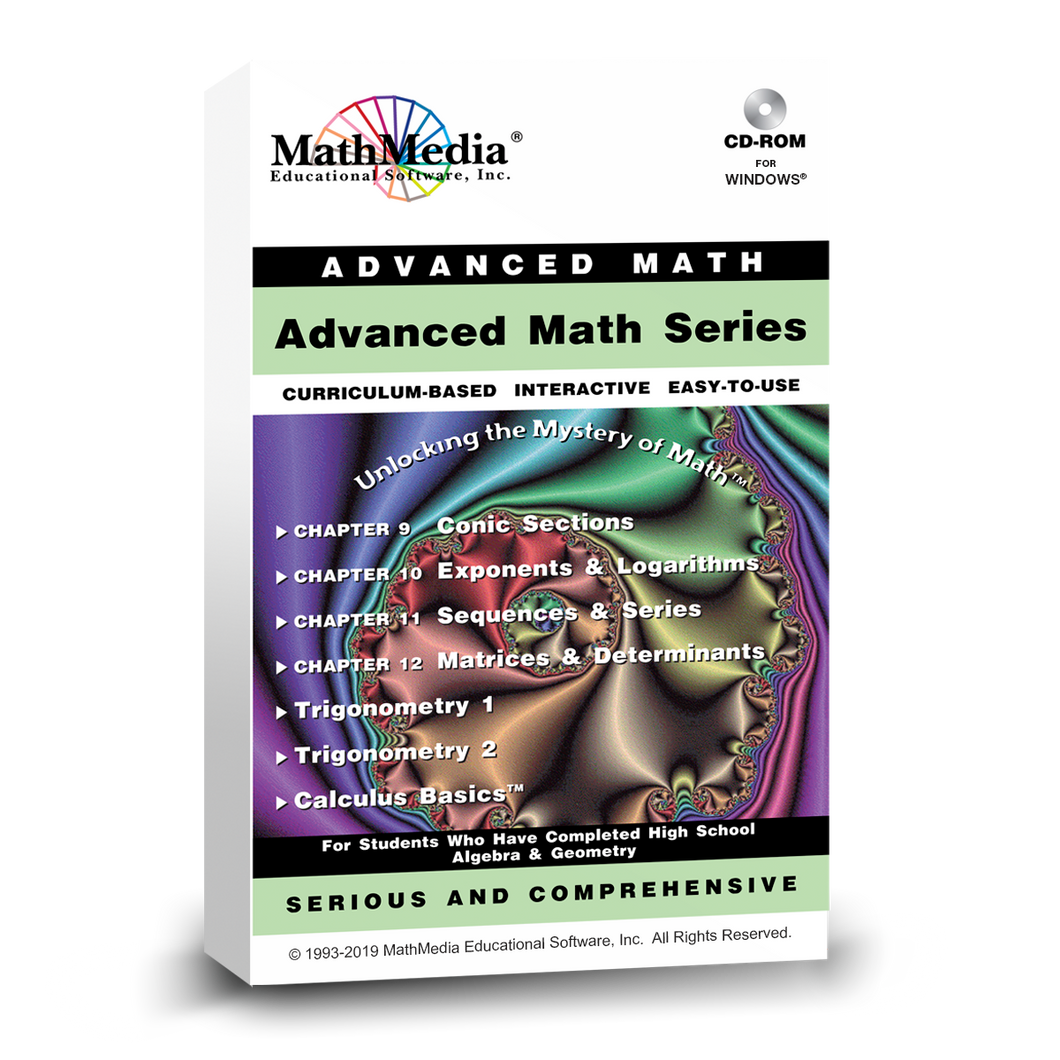Regular price \$349.00 \$87.25 Sale

Curriculum Description:
The Advanced Math Series is a set of 7 instructional software programs designed for advanced math high school students and college algebra students. The first four programs, Chapters 9 – 12, are a continuation of the Algebra By Chapter Series, set of 8 Chapters, and complete the high school Algebra 2 curriculum. Trigonometry 1 and 2 provide a complete Trig course (high school and college). Calculus Basics begins with comprehensive lessons on pre-calculus and then covers the calculus curriculum on derivatives.

All these pre calculus lessons contain step-by-step pre calculus and calculus basics help. Graphing calculator lessons are scattered throughout the 7-program series and help students keep up with today's math education usage of graphing calculators.

Advanced Math Series Curriculum SyllabusPrint Product DescriptionsUseful for Grant Writers

The Advanced Math Series includes 7 Chapters:

CH 9: Conic Sections
CH 10: Exponents & Logarithms
CH 11: Sequences & Series
CH 12: Matrices & Determinants
Trigonometry - Part 1 and Part 2
Calculus Basics - Derivatives

A comprehensive list of subject matter for each chapter can be found by clicking on the respective links below.

The first four programs in this mathematics education software series are a continuation of the Algebra by Chapter Series and is appropriate for Intermediate Algebra and Pre Calculus students. The Conic Section program provides in-depth practice and understanding of calculating and graphing conic sections. The Exponent and Logarithm program teaches beginning through advanced exponents with log functions calculated both by hand and by calculator. Sequences and Series provide interactive practice with formulas leading to the more advanced topics of binomial expansions. Understanding and using Matrices and Determinants teaches the rules for solving multiple equations simultaneously, providing guided practice along the way. Each of these math education programs has a word problem section and concludes with a comprehensive test of the material learned in the program.

These advanced mathematics software programs contain Trigonometry 1 and 2 which together form a complete course and can be used to prepare for college math placement exams, to use as a resource while in a Trig course, or use to test out of college Trig with the CLEP exam. Pre-Calculus help is available in each of these software titles. Concentrated learning of Trigonometric functions provides the student with ample practice to form a solid understanding of Trig. Each of these two Trig programs concludes with a 30-question test with hints and complete solutions accessed upon student request. This mathematics education software series concludes with Calculus Basics which contains a comprehensive pre calculus learning section and then teaches the basics of calculus (derivatives).

Assessments
Each chapter concludes with a comprehensive test of the material covered.

These programs automatically “bookmark” the student’s last viewed screen and will prompt the student to return to that screen upon their return.

FEATURES AT A GLANCE:
- Easy to Use
- Plenty of Practice Screens with on-demand help
- Chapter Tests
- Each assessment is scored
- Bookmarking

Who it’s for:
This intermediate algebra, precalculus, and trig software is appropriate for students in High School through College Algebra, Trigonometry, Pre-Calculus, and Calculus Basics courses. Use this software as a supplemental resource for your class work or use it as a standalone complete comprehensive curriculum.

PreRequisites:
Algebra Series By Chapter